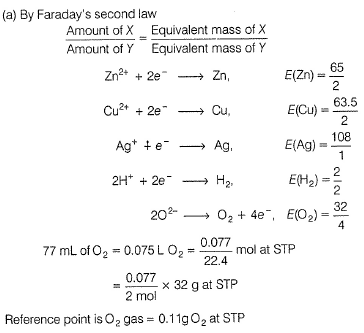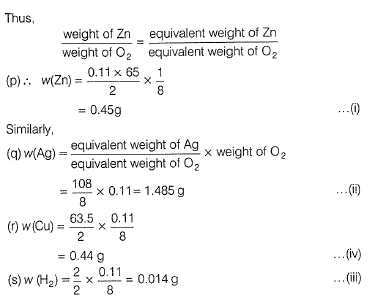Courses

# Test: Electrolysis And Faraday's Laws

## 32 Questions MCQ Test Chemistry Class 12 | Test: Electrolysis And Faraday's Laws

Description
This mock test of Test: Electrolysis And Faraday's Laws for Class 12 helps you for every Class 12 entrance exam. This contains 32 Multiple Choice Questions for Class 12 Test: Electrolysis And Faraday's Laws (mcq) to study with solutions a complete question bank. The solved questions answers in this Test: Electrolysis And Faraday's Laws quiz give you a good mix of easy questions and tough questions. Class 12 students definitely take this Test: Electrolysis And Faraday's Laws exercise for a better result in the exam. You can find other Test: Electrolysis And Faraday's Laws extra questions, long questions & short questions for Class 12 on EduRev as well by searching above.
QUESTION: 1

### Only One Option Correct Type This section contains 16 multiple choice questions. Each question has four choices (a), (b), (c) and (d), out of which ONLY ONE is correct Q. During the electrolysis of aqueous Zn(NO3)2 solution

Solution: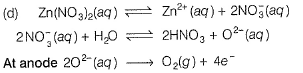In preference to reduction of Zn2+ at cathode, O2- (aq) is oxidised to O2 at anode.

QUESTION: 2

### Schematic diagram of an electrolytic cell is

Solution: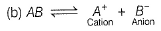In electrolytic cell,
Cation moves to cathode and anion moves to anode.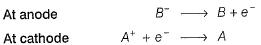Electrons move anode to cathode.

QUESTION: 3

### The metal that can not be obtained by electrolysis of an aqueous solution of its salt is [JEE Main 2014]

Solution:

In electro chemical series (ECS),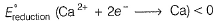thus, Ca2+ ion is not reduced to C a instead, H2O is reduce d to H2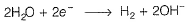Ag, Cu and Cr are below H in ECS (E°reduction > 0), thus they are reduced to metals on electrolysis.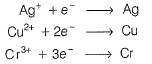Note In ECS, Ca, H, Cr, Cu elements lying above H are better reducing agents thus are easily oxidised.

QUESTION: 4

When during electrolysis of a solution of AgNO3 ,9650 C of charge pass through the electroplating bath,the mass of silver deposited on the cathode will be

Solution: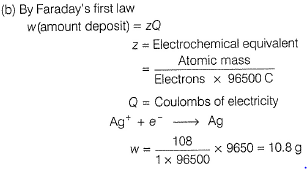QUESTION: 5

Refining of impure metal is done by electrolysis using impure metal as anode.Select the correct statement about this refining.

Solution:

When impure metal (M) is anode and pure metal is made cathode, then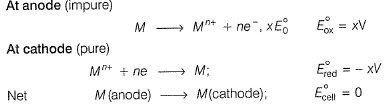Thus, impure metal from the anode is deposited at the cathode.

QUESTION: 6

W g of Ag is deposited at the cathode of one electrolytic cell due to passage of 1A of current for 1h.Time required for passage of current to deposit W g of Mg by the same value of current is

Solution: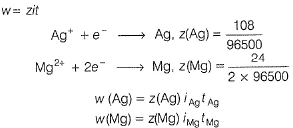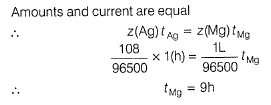QUESTION: 7

In the refining of silver by electrolytic method, current of 5A is passed for 2 h using 100 g of impure anode of silver (of 95% purity). Weight of silver anode after electrolysis is

Solution:

By Faraday 's first law of electrolysis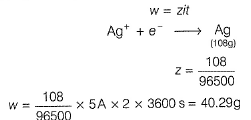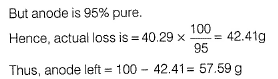QUESTION: 8

In the electrorefining of metals, impure metal is

Solution:

Impure metal is made the anode and oxidation takes place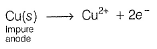Cu2+ formed are reduced at cathode and thus, deposited there.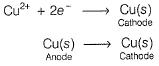QUESTION: 9

In the electrosynthesis,potassium manganate (VII) is converted to manganese(IV) dioxide. By passage of 1F of electrolysis ,one mole of potassium manganate(VII) will form manganese dioxide.

Solution:

Manganate (VII) is MnO4- with ON of Mn = + 7
1 mole required 3F of electricity thus, 1 F will reduce only (1/3) mole MnO4- t o MnO2.
Thus, MnO2 formed = 0.33 mol.

QUESTION: 10

When Al2O3 is electrolysed ,cation and anions are discharged. For a given quantity of electricity,ratio of number of moles of Al and O2 gas is

Solution:

When same quantity of electricity is passed, elements/gases are formed in the ratio of their equivalents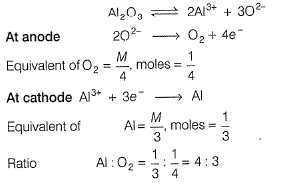.

QUESTION: 11

1 Faraday of electricity is passed through the solution containing 1 mole each of AgNO3, CuSO4, AlCland SiCl4. Elements are discharged at the cathode.Number of moles of Ag, Cu,Al and Si formed will be in the ratio of

Solution:

(d)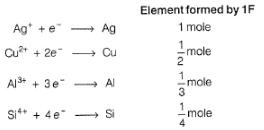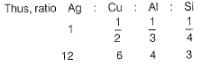QUESTION: 12

During electrolysis of acidified water ,O2 gas is formed at the anode. To produce O2 gas at the anode at the rate of 0.224 cc per second at STP,current passed is

Solution: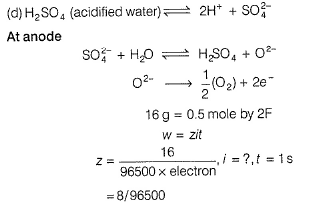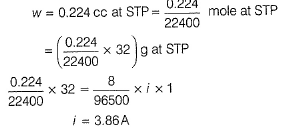QUESTION: 13

A solution of copper(II) sulphate (VI) is electrolysed between copper electrodes by a currrent of 10.0 A for exactly 9650 s.Which remains unchanged?

Solution: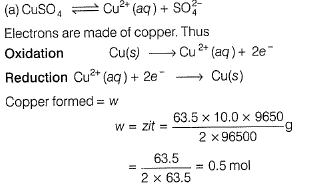0.5 mole of copper is dissolved from the anode. Thus, its mass decreases. 0.5 mole of copper from the anode is deposited at the cathode. Thus, its mass increases.
Thus, molar concentration of aqueous solution of CuSO4 remains unchanged.

QUESTION: 14

A 300 mL solution of NaCl was electrolysed for 60.0 min. If the pH of the final solution was 12.24,average current used is

Solution: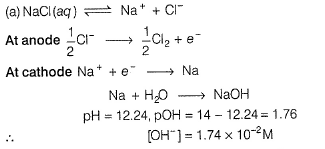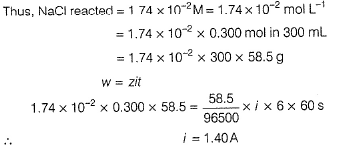QUESTION: 15

100 mL of a buffer of 1 M NH3(aq) and 1 M NH4+(aq) are placed in two volatic cells separately.A current of 1.5 A is passed through both cells for 20 min. If electrolysis of water only takes place

2H2O +O2 + 4e- → 4OH- (RHS

2H2O  → 4H+. + O2 + 4e- (LHS)

then pH of the

Solution: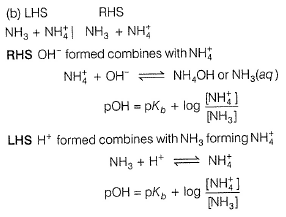In RHS, [NH3] increases hence, pOH decreases
pH = 14 - pOH
Hence, pH of RHS increases.
In LHS, [NH4+] increases, hence pOH increases and pH decreases.

QUESTION: 16

What product are formed during the electrolysis of a concentrated aqueous solution of sodium chloride using an electrolytic cell in which electrodes are separated by a porous pot?

I. Cl2(g)
II. NaOH(aq)
III. H2(g)
IV. NaClO(aq)
V. NaClO3(aq)

Select the correct choice.

Solution: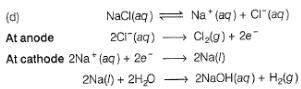Cl2(g) is formed at anode and NaOH and H2 are formed at cathode. Since, cathode and anode are separated. Hence. there is no reaction between the products formed at the cathode and anode.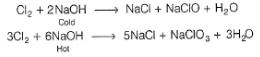Note: but these reaction do not occur

Note: NaCl is neutral pH = 7 initially. After electrolysis , NaOH is formed

Hence, solution become, basic pH >7

*Multiple options can be correct
QUESTION: 17

One or More than One Options Correct Type

This section contains 5 multiple type questions. Each question has 4 choices (a), (b), (c) and (d), out of which ONE or MORE THAN ONE are correct.

Select the correct point(s) of distinction between a volatic cell and electrolysis cell.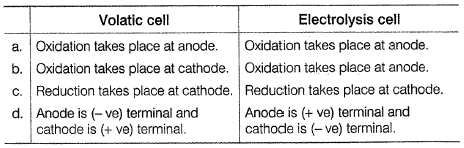Solution:

(a.c.d) Regardlessvehether a cell is a volatic or an electrolysis cell, anode s the electrode at which oxidation takes place and cathode is the electrode at which reduction takes place. In electrochemical cell, Zn → Zn2+ + 2e- .

It is losing electrons thus, anode is negative terminal. In electrolysis cell. cation migrates to cathode thus -ye terminal. anion migrates to anode thus +ve terminal.

*Multiple options can be correct
QUESTION: 18

In the electrolysis of aqueous sodium chloride solution, two types of reactions can take place at anode :

I. 2Cl- (aq) → Cl2(g) +2e-

II. 2H2O(l)g → O2 (g) + 4H+(aq) + 4e-

Select the correct statement(s) about these.

Solution: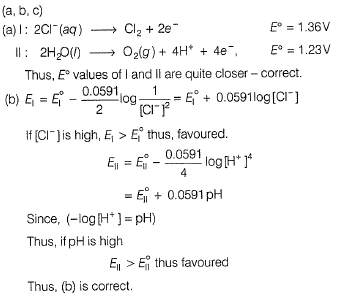(c) Experrnent indicates that the applied voltage required is always greater than the voltage calculated from the standard E0ox or E0red. The additional voltage required is called overvoltage. Due to this, Cl2 and not O2 s formed. This, (c) 6 correct.

*Multiple options can be correct
QUESTION: 19

Select the correct statement(s) about electrolysis of aqueous CuSO4 solution.

Solution:

(a)

Oxidation takes place at anode - correct

2H2O(l) →4H+ (aq) + O2(g) + 4e-

(b) Reduction take place at cathode - correct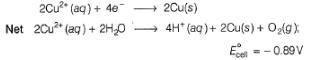since, E0cell < 0, in electrolysis.

Cell reaction is spontaneous.

(c) Copper is deposited at the cathode, hence the meted to be electroplated (say Fe), should be made cathode - correct (d) Since. Cu2+(aq)- (blue) is reduced Cu(s), hence blue colour fades - correct.

*Multiple options can be correct
QUESTION: 20

Select the correct observation about electrolysis.

Solution: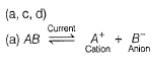Thus, electric current is used to carry out a non-spontaneous reaction.
Thus. (a) is correct.
(b) Since reactions are reverse of electrochemical cell, hence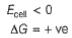thus, (b) is incorrect

(c) cation goes to cathode and anion goes to anode and are discharged. Thus, correct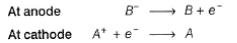(d) some additional voltage called overvoltage is set up and thus other reactions also take place. thus correct

*Multiple options can be correct
QUESTION: 21

Which pair of electrolysis could be distinguished by the products of electrolysis using inert electrodes?

Solution:

a(Cu So4 and CuCl2 changes to Cu and solution becomes colourless

(b) KCl changes to Cl2 (yellow)
KI changes to I2 (voilet)

Thus, distinguished.

(c) AgNo3 No change

CuSo4 (blue) changes to colourless

(d) CuBr2 (blue) both changes to colourles.

NiBr2 (Blue)

QUESTION: 22

Comprehension Type

This section contains a passage describing theory, experiments, data, etc. Two questions related to the paragraph have been given. Each question has only one correct answer out of the given 4 options (a), (b), (c) and (d)

Passage I

A constant current of 30 A is passed through an aqueous solution of NaCl for a time of 1.00 h.

Thus NaOH formed due to electrolysis is

Solution:
QUESTION: 23

Passage I

A constant current of 30 A is passed through an aqueous solution of NaCl for a time of 1.00 h.

Thus Cl2 formed under STP condition is

Solution:

At anode 2Cl- —-±>Cl2+2e-
At cathode 2Na+ +2e- ——-> 2Na.
So 2 Faraday of electricity is required to liberate 1 mole = 22.4 liters of Cl2 at STP.
Amount of current passed
= 30 x 60 x 60=108000 coulombs.
So 108000 coulombs will liberate =108000/(96500 x2) = 0.5596 moles Cl2.
No.of liters of Chlorine liberated at STP
= 0.5596 x 22.4 = 12.53 liters.

QUESTION: 24

Passage II

In hydrogen economy fuel-cell,anodic and cathodic reactions are

Anodic

H2+2OH- → 2H2O + 2e-

Cathodic

O2+2H2O + 2e- → 4OH-

67.2 L H2 at STP react in 15 min.Entire current is used for electro deposition of copper from copper(II) sulphate solution. Average current produced in fuel cell is

Solution: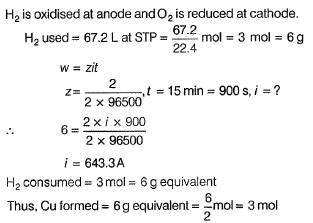QUESTION: 25

Passage II

In hydrogen economy fuel-cell,anodic and cathodic reactions are

Anodic

H2+2OH- → 2H2O + 2e-

Cathodic

O2+2H2O + 2e- → 4OH-

67.2 L H2 at STP react in 15 min.Entire current is used for electro deposition of copper from copper(II) sulphate solution. Copper deposited would be

Solution:*Answer can only contain numeric values
QUESTION: 26

One Integer Value Correct Type

This section contains 6 questions, when worked out will result in an integer value from 0 to 9 (both inclusive)

Mn+ solution was electrolysed for 75 min by a current of 0.30 A. Mass of the metal M deposited at the cathode was 9.958 g.Mn+ contains 68 protons and 69 neutrons. What is the value of n?

Solution: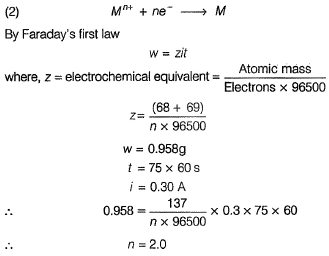*Answer can only contain numeric values
QUESTION: 27

An electrochemical cell was based on the following reaction:

Mn(OH)2(s) + H2O2(aq) → MnO2(s) + 2H2O (e)

During the opeartion of this for 1 min, 0.135 g of MnO2 was produced. What is the average electric current (in ampere ) produced by the cell?

Solution: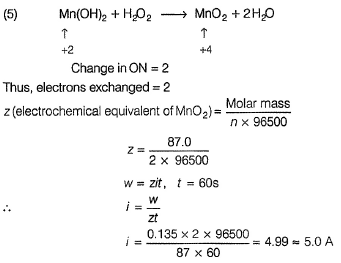*Answer can only contain numeric values
QUESTION: 28

In a fuel cell, following reactions takes place and electricity is produced.

Anodic

H2+2OH- → 2H2O + 2e-

Cathodic

O2+2H2O + 4e- → 4OH-

If 100.8 L of H2 at STP reacts in 96500 s,what is the average current produced (in ampere)?

Solution: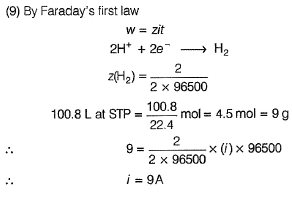*Answer can only contain numeric values
QUESTION: 29

How long (in minutes ) a current of 1.56 A has to be passed through a solution of AgNO3 to coat a metal surface of 80 cm2 with a 0.010 mm thick layer? Density of silver is 10.5 g cm-3.

Solution: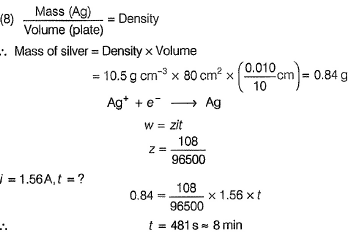*Answer can only contain numeric values
QUESTION: 30

A fully charged battery contains 500 mL of 5.00 M H2SO4. What is the concentration of H2SO4 in the battery after 6.0 A of current is drawn from the battery for 13.40 h?

Solution: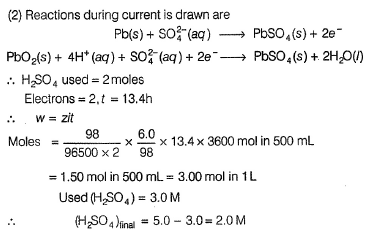*Answer can only contain numeric values
QUESTION: 31

A hydrogen-oxygen fuel cell opeartes on the simple reaction: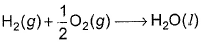If the cell is designed to produce 1.46A of current and if the H2 is contained in a 1.0 L tank at 2.00 atm pressure at 298K, how long can the fuel cell operate(in hour) before the H2 runs out.(Assume unlimited supply of oxygen)

Solution: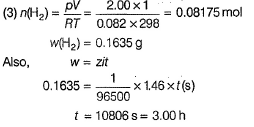QUESTION: 32

Matching List Type

Choices for the correct combination of elements from Column I and Column II are given as options (a), (b), (c) and (d), out of which one is correct

Four electrolytic cells containing solution of ZnSO4, AgNO3, CuSO4 and dil. H2SO4 are connected in series. A steady current of 1.5 A was passed through them until 77 mL of O2 gas at STP is formed in anode compartment of H2SO4 solution. Match the different elements/gas formed In Column I with their values in Column II and select the answer from the codes given below. (Cu = 63.5, Zn = 65, Ag = 108, S = 32, H = 1, 0 = 16)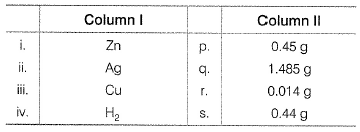Codes   i       ii      iii      iv

Solution: